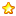﻿ 把發車音樂配上歌詞 - 其他討論題目 (N) - hkitalk.net 香港交通資訊網 - Powered by Discuz!

##用戶名 Email 自動登錄 找回密碼 密碼 會員申請加入
Custom Search
﻿

 (B0)香港巴士車務及車廂設備 (B1)香港巴士廣告消息/廣告車行踪 (B2)香港巴士討論 (B3)巴士攝影作品貼圖區 (B3i)即拍即貼 -手機相&翻拍Mon相 (B4)兩岸三地巴士討論 (B5)外地巴士討論 Advertisement (B6)旅遊巴士及過境巴士 (B7)巴士特別所見 (B11)巴士精華區 (B22)巴士迷吹水區 (V)私家車,商用車,政府及特種車輛 (A6)相片及短片分享/攝影技術 (A10)香港地方討論 (A11)消費著數及飲食資訊 (A16)建築物機電裝置及設備 (A19)問路專區 (N)其他討論題目 (F1)交通路線建議 (C2)航空 (C3)海上交通及船隻 (D1)公共交通有關商品 (Y)hkitalk.net會員福利部 (Z)站務資源中心 (R1)香港鐵路 (R2)香港電車 (R3)港外鐵路 (O1)omsi討論區 (O2)omsi下載區 (O3)omsi教學及求助區 (M1)小型巴士綜合討論 (M2)小型巴士多媒體分享區 (M3)香港小型巴士字軌表 最新板務資訊# 把發車音樂配上歌詞

3345iPower 22HugeC

Level 23353發表於 2018-12-18 07:57 | 顯示全部樓層 |閱讀模式

 不少在日本的鐵道迷也會把發車音樂配上歌詞，我現在也開始嘗試，但配上的歌詞是來自IGCSE（9-1）物理科的課本。 以下是我的一些作品： 1. （原曲：東武鐵道-Passenger） Work is defined as energy transferred to objects by a force which is when a force exerts on objects over a displacement Parallel to the force and it has the following ratio that work done equals to force times the object's displacement 2. （原曲：夕焼け小焼け）（八王子1-6台） As work done can convert to kinetic energy, so it is half of mass times velocity square 3. （原曲：たき火）（豐田1-4台） When a force moves mass upwards in constant velocity, then there is no kinetic energy gained from it Then potential energy is work done by the force, which equals force times displacement called as mgh 4. （原曲：熊谷市歌B）（籠原1-2台） Power's the rate when work's done or energy transferred, it can be found by work done or energy over time 5. （原曲：上を向いて歩こう）（友部1台） Mechanical energy's sum of kinetic energy and potential energy combined together, When no external work is done, mechanical energy's conserved 6.  （原曲：スプリングボックス）（池袋7-8台） Efficiency is useful energy output over total input times hundred percent 7. （原曲：JR東日本特急車內提示音1） Pressure is defined as normal force exerted on a surface per unit area
Cathay 289 cleared for takeoff on runway
 本版積分規則 回帖後跳轉到最後一頁